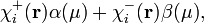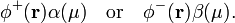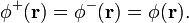# Electron orbital

In quantum chemistry, the term electron orbital (or more often just orbital) is synonymous to one-electron function, i.e., a function of a single vector r, the position vector of a given electron. The majority of quantum chemical methods expect that an orbital has a finite norm, i.e., that the orbital is normalizable (quadratically integrable), and hence this requirement is often added to the definition.

In other branches of chemistry than computational quantum chemistry, an orbital is often seen as a wave function of a single electron, meaning that an orbital is seen as a solution of an effective and physically relevant one-electron Schrödinger equation. Such one-electron Schrödinger equation is often of the Hartree-Fock type. This point of view is a specialization of the quantum chemical definition, since obviously solutions of one-electron Schrödinger equations are one-electron functions, i.e., orbitals. In the past quantum chemists, too, restricted the term "orbital" to solutions of certain one-electron Schrödinger equations, giving orbitals physical meaning. This usage disappeared from quantum chemistry, resulting in the present definition of an orbital as the purely mathematical construct: "normalizable one-electron function". In the present quantum chemical usage an orbital may—or may not—be an eigenfunction of a one-electron Hamiltonian (that is, a solution of a one-electron Schrödinger equation).

Usually one distinguishes two kinds of orbitals: atomic orbitals and molecular orbitals. Atomic orbitals are expressed with respect to one Cartesian system of axes centered on a single atom. Molecular orbitals (MOs) are "spread out" over a molecule. Usually this is a consequence of an MO being a linear combination (weighted sum) of atomic orbitals centered on different atoms.

## Definitions of orbitals

Several kinds of orbitals can be distinguished.

### Atomic orbital (AO)

See the article atomic orbital

### Molecular orbital (MO)

See the article molecular orbital

### Spinorbitals

The AOs and MOs defined so far depend only on the spatial coordinate vector r of a single electron. In addition, an electron has a spin coordinate μ, which can have two values: spin-up or spin-down. A complete set of functions of μ consists of two functions only, traditionally these are denoted by α(μ) and β(μ). These functions are eigenfunctions of the z-component sz of the spin angular momentum operator with eigenvalues ±½.

#### Spin atomic orbital

The most general spin atomic orbital of an electron is of the form$\chi_i^{+}(\mathbf{r})\alpha(\mu) + \chi_i^{-}(\mathbf{r})\beta(\mu),$

where r is a vector from the nucleus of the atom to the position of the electron. In general this function is not an eigenfunction of sz. More common is the use of either$\chi_i(\mathbf{r})\alpha(\mu) \quad\hbox{or}\quad \chi_i(\mathbf{r})\beta(\mu),$

which are eigenfunctions of sz. Since it is rare that different AOs are used for spin-up and spin-down electrons, we dropped the superscripts + and −.

#### Spin molecular orbital

A spin molecular orbital is usually either$\phi^{+}(\mathbf{r})\alpha(\mu) \quad\hbox{or}\quad \phi^{-}(\mathbf{r})\beta(\mu) .$

Here the superscripts + and − might be necessary, because some quantum chemical methods distinguish the spatial wave functions of electrons with different spins. These are the so-called different orbitals for different spins (DODS) (or spin-unrestricted) methods. However, many quantum chemical methods apply the spin-restriction:$\phi^{+}(\mathbf{r}) = \phi^{-}(\mathbf{r}) = \phi(\mathbf{r}).$

Chemists express this spin-restriction by stating that two electrons [an electron with spin up (α), and another electron with spin down (β)] are placed in the same spatial orbital φ. This means that the total N-electron wave function contains a factor of the type φ(r1)α(μ1)×φ(r2)β(μ2).

## Applications

Before the advent of electronic computers, orbitals (molecular as well as atomic) were used extensively in qualitative arguments explaining properties of atoms and molecules. Orbitals still play this role in introductory texts and also in organic chemistry, where orbitals serve in the explanation of some reaction mechanisms, for instance in the Woodward-Hoffmann rules. In modern computational quantum chemistry the role of atomic orbitals is different; they serve as a convenient expansion basis, comparable to powers of x in a Taylor expansion of a function f(x), or sines and cosines in a Fourier series.

### Atomic orbitals

Originally AOs were defined as approximate solutions of atomic Schrödinger equations, see the section solution of the atomic Schrödinger equation in atomic orbital for more on this. Very often atomic orbitals are depicted in polar plots. In fact, the angular parts (functions depending on the spherical polar angles θ and φ) are commonly plotted. The angular parts of atomic orbitals are functions known as spherical harmonics.

As stated, quantum chemists see AOs simply as convenient basis functions for quantum mechanical computations. See the section AO basis sets in the article atomic orbital for more on the use of atomic orbitals in quantum chemistry. See Slater orbital for the explicit analytic form of orbitals and see Gauss type orbitals for a discussion of the type of AOs that are most frequently used in computations.

### Molecular orbitals

To date the most widely applied method for computing molecular orbitals is a Hartree-Fock method in which the MO is expanded in atomic orbitals. Since the term "Hartree-Fock" (HF) is in quantum chemistry almost synonymous with the term "self-consistent field" (SCF), such an MO is often referred to as an SCF-LCAO-MO.

See the section computation of molecular orbitals in molecular orbital for a simple example of a case where an MO is almost completely determined by symmetry. In more complicated cases it is necessary to solve the Hartree-Fock-Roothaan equations, which have the form of a generalized eigenvalue problem. The dimension of this problem is equal to the number of atomic orbitals included in the AO basis (see AO basis sets in atomic orbital for more details).

## History of term

Orbit is an old noun introduced by Johannes Kepler in 1609 to describe the trajectories of the earth and the planets. The adjective "orbital" had (and still has) the meaning "relating to an orbit". When Ernest Rutherford in 1911 postulated his planetary model of the atom (the nucleus as the sun, and the electrons as the planets) it was natural to call the paths of the electrons "orbits". Bohr used the word as well, although he was the first to recognize (1913) that an electron orbit is not a trajectory, but a stationary state of the hydrogen atom.

After Schrödinger (1926) had solved his wave equation for the hydrogen atom (see hydrogen-like atoms for details), it became clear that the electronic orbits did not resemble planetary orbits at all. The wave functions of the hydrogen electron are time-independent and smeared out; they are more like unmoving clouds than planetary orbits. As a matter of fact, the angular parts of the hydrogen wave functions are spherical harmonic functions and hence they have the same appearance as these functions. (See spherical harmonics for a few graphical illustrations).

In the 1920s electron spin was discovered, whereupon the adjective "orbital" started to be used in the meaning of "non-spin", that is, as a synonym of "spatial". In scientific papers of around 1930 one finds discussions about "orbital degeneracy", meaning that the spatial (non-spin) parts of several one-electron wave functions have the same energy. Also the terms orbital- and spin-angular momentum date form these days.

In 1932 Robert S. Mulliken coined the noun "orbital". Mulliken wrote:

From here on, one-electron orbital wave functions will be referred to for brevity as orbitals.

Note that here, evidently, Mulliken uses "orbital" as relating to "spatial" (non-spin) and defines an orbital as what is now called a "spatial orbital". Then Mulliken went on in the same article to distinguish atomic and molecular orbitals.

Later the somewhat unfortunate term "spinorbital" was introduced for the product functions φ(r)α(μ) and φ(r)β(μ) in which φ(r) has the tautological name "spatial orbital" and α(μ) and β(μ) are called "one-electron spin functions". The term "spinorbital" is unfortunate because it merges in one word the concepts of spin and orbital, which were distinguished carefully by early writers on quantum mechanics. For instance, one of the pioneers of theoretical chemistry, Walter Heitler, juxtaposes two-electron spin functions and two-electron orbital functions. In the phrase "two-electron orbital function", Heitler uses orbital as an adjective synonymous with spatial (non-spin). Note, parenthetically, that Heitler does not refer to a "two-electron orbital", (there is no such thing as a two-electron orbital !) and that an inexperienced reader may easily—and erroneously—interpret the term "two-electron orbital function" as "two-electron orbital".

## References and notes

1. Here "orbital" is used as a noun. In quantum mechanics, the adjective orbital is often used as a synonym of "spatial" (as in orbital angular momentum), in contrast to spin (as in spin angular momentum). See the section History of term in this article for more on this.
2. R. S. Mulliken, Electronic Structures of Molecules and Valence. II General Considerations, Physical Review, vol. 41, pp. 49-71 (1932)
3. W. Heitler, Elementary Wave Mechanics, 2nd edition (1956) Clarendon Press, Oxford, UK.